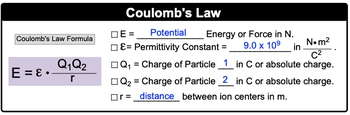## General Chemistry

Learn the toughest concepts covered in Chemistry with step-by-step video tutorials and practice problems by world-class tutors

11. Bonding & Molecular Structure

# Coulomb's Law

Coulomb's Law is used to determine the forces experienced by charged particles.

Coulomb's Law
1
concept

## Coulomb's Law Concept 11m
Play a video:
now columns law is used to determine the attractive and repulsive forces between a pair of charged particles. Now using columns. Law formula allows you to determine the potential energy between the particles. Now we're going to say that potential energy is directly proportional to the charges of the particles, meaning the larger. The charge is the largest of potential energy. But potential energy is inversely proportional to the distance between the particles. So basically, the larger the distance between the particles will lower, their potential energy will be. And what we need to realize here is that the greater the potential energy than the stronger the Ionic bond between our opposed charges will be or charged particles will be. So just remember these fundamental theoretical ideas behind Klum's law. Click on the next video and let's investigate Combs law formula.
2
concept

## Coulomb's Law Concept 21m
Play a video:
with columns. Law, We have our columns law formula where e. Which is our potential energy or force in Newtons. In physics, you would see this variable as 1/4 pi. Same vary variable with sub not but for simplicity. I'm just having it. Is that single variable here? We're going to say that it is in place off what we call the permitted constant, which is 9.0 times 10 to the nine Newtons. Times meter squared over Coolum squared Q one and Q two represent our particles one and two that are in columns or absolute charge. Our, which down here would actually be r squared equals the distance off the ion centers in meters. Not finally, just remember that the absolute charge of an ion is equal to 1.602 times 10 to the negative 19 cool apps. That would mean that if you had a charge of, let's say, plus two, that's your absolute charge. But how would you convert it to cool them? You will just multiply by that same value, and that would be 3.204 times 10 to the negative Coolio's so now that we know comes law formula. Let's move on to the next video and take a look at the example question.3
example

## Coulomb's Law Example1m
Play a video: